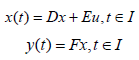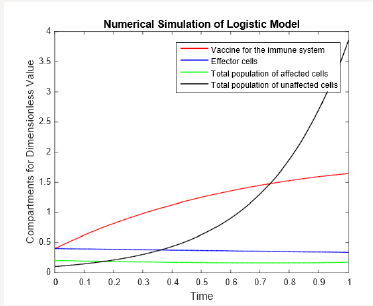• Submissions# A Control of Logistic Model of Bladder Cancer with Dimensionless Estimate Parameters

1 Department of Mathematics and Statistics, University of Lahore, Pakistan

2 Department of Allied Health Sciences (DPT), University of Lahore, Pakistan

*Corresponding author: Muhammad Farman, Department of Mathematics and Statistics, Pakistan

Submission: December 12, 2018;Published: January 09, 2019

DOI: 10.31031/ETUN.2019.02.000544ISSN 2578-0395
Volume2 Issue4

#### Abstract:

In this manuscript, Immunotherapy with Bacillus Calmette Guerin (BCG) vaccine is used for the treatment of superficial bladder cancer. Considered the cancer model regarding tumor- immune interactions in the bladder as a result of BCG therapy having four variables, B, E, Ti and Tu represents the vaccine for the immune system, effector cells, the total population of affected and unaffected cells respectively. Controllability and observability are treated for logistic model according to dimensionless estimate parameters values. Also numerical simulation are carried out to show the actual behavior of the system.

Keywords: Controllability; Observability; BCG immunotherapy; Numerical simulations

#### Introduction

Cancer is a class of diseases characterized by out-of-control cell growth. More dangerous, or malignant, tumors form when two things occur: 1) a cancerous cell manages to move throughout the body using the blood or lymphatic systems, destroying healthy tissue in a process called invasion. 2) That cell manages to divide and grow, making new blood vessels to feed itself in a process called angiogenesis. When a tumor spreads and invades to the body it destroys other healthy tissues, know as metastasized. This process is itself called metastasis; this stage is difficult to cure. According to the American Cancer Society, Cancer is the second most common cause of death in the US and accounts for nearly 1 of every 4 deaths .

#### Mathematical Model

We describe the interaction between tumor cells within the bladder, the immune system, and the BCG immunotherapy with a system of nonlinear ordinary differential equations. In our model the tumor cells are divided into two subpopulations; those that have been infected (Ti) with BCG (B) and those that are still uninfected Tu and susceptible. As the tumor cells are divided into two pools, the total number of tumor cells is given by: T=Ti +Tu. Since effector cells (E) target and destroy infected tumor cells (Ti) the latter must decrease at an intensity that is proportional to their encounter. By taking random mixing as a first approximation, the encounter rate is proportional to the product p3ETi, where p3 is a rate constant. Similarly, tumor cells become infected with BCG at a rate proportional to the product p2 BTu where p2 is a rate coefficient. Thus the dynamics of the individual pools of tumor populations, the tumor growth factor in this model is given by:where γ is the growth rate of tumor cells, and β is the death of tumor cells as a result of self-limiting competition for resources such as oxygen and glucose. β-1 may be viewed as the maximum carrying capacity of the tumor under logistic growth [4-6].The parameters values of exponential model and logistic model for human are given in the table. Note that dimensionless estimates are obtained form source value using transformation .

#### Controllability and Observability

A mathematically linear control system is given by the following two equationswhere x(t)∈Rn , u(t)∈Rp and y(t)∈Rk for t∈I . I is closed interval. Elements of matrices are in L2 (I, R).u(⋅)∈L2(I, Rp ) is called input/control, y(⋅)∈L2(I, Rk ) is called output and x(t)∈Rn , t∈I is called state [7-9,10]. If we consider the BCG immunotherapy in the bladder is the only input and concentration of BCG in effector cell is only measured output then E = [1 0 0 0]T, and F = [0 1 0 0] The controllability and observability matrix areThe rank of controllability and observability matrices is 1. The only measured output is affected cell due to cancer that we can easily measure. The system is not controllable and neither observable. The logistic model after substituting the dimensionless values, we getThe equilibrium points of (B, E, Ti, Tu) are (0.0701753, 1.70046, 1.48658, 139.033), (3, 5.56913×10−16, 4.39104×10−16, 0) and lies in the feasible region so the system is stable. In this case, the rank of controllability and observability matrices are 4 respectively. Hence the system is controllable and observable.

The mathematical analysis of Cancer model with nonlinear incidence has been presented. To observe the effects of the parameters using in this dynamics of Cancer model (1)-(4), conclude several numerical simulations varying the value of parameters given in Table 1 for source value and dimensionless values. Figure 1-4 shows by increasing the vaccination of immune system for logistic model according to dimensionless values, effecter cells and total population of affected cells become stable while total population of unaffected cells increases efficiently.

Table 1:Table of parameters values used in the model.Figure 1:Numerical simulation of logistic model with dimensionless values.Figure 2:Numerical simulation of logistic model with dimensionless values.Figure 3:Numerical simulation of logistic model with dimensionless values.Figure 4:Numerical simulation of logistic model with dimensionless values.#### Conclusion

We find the linear control of the model because if the linear system is controllable and observable then nonlinear system may or may not be controllable and observable. Model consist two categories according to parameter values i.e source and dimensionless values. For dimensionless values of the parameters, we have two equilibrium points in which system has solution in the feasible region. Model is controllable and observable for first equilibrium point but for second equilibrium point model is partially controllable and observable. Numerical simulations reveals that the logistic model give better impact with BCG vaccination strategies. We observe that BCG has a very positive impact to control cancer at the initial level.

﻿

We at Crimson Publishing are a group of people with a combined passion for science and research, who wants to bring to the world a unified platform where all scientific know-how is available read more...